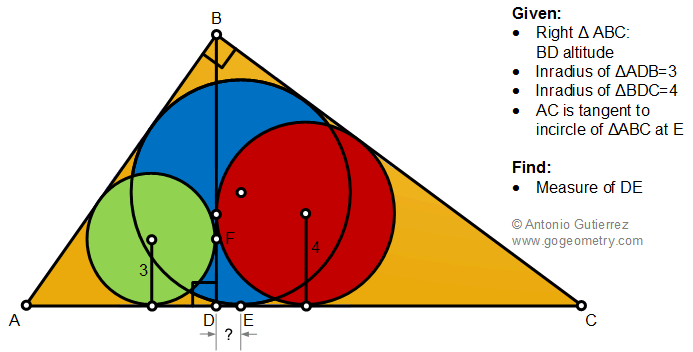# Geometry Problem 1486 with Solution: Right Triangle, Altitude, Incircle, Tangent, Measurement

The figure shows a right triangle ABC with altitude BD and AC tangent to the incircle at E. If the measure of inradii of triangles ADB and BDC are 3 and 4, respectively, find the measure of DE.#### Incircle

Incircle is a circle within a triangle, that is tangent to each side.